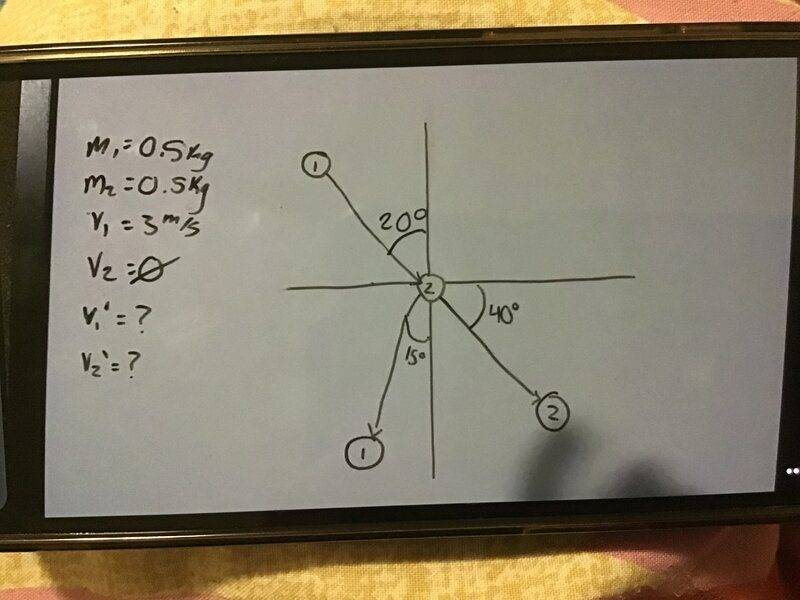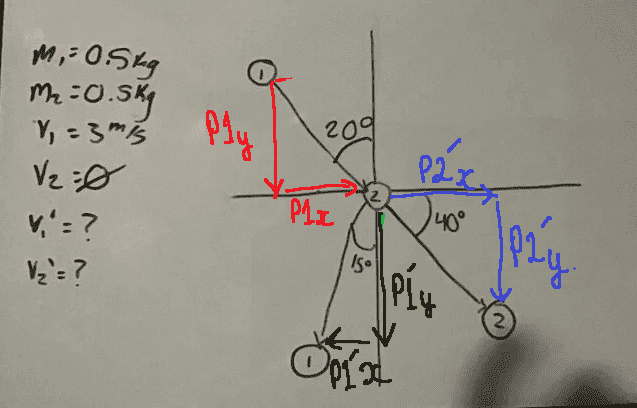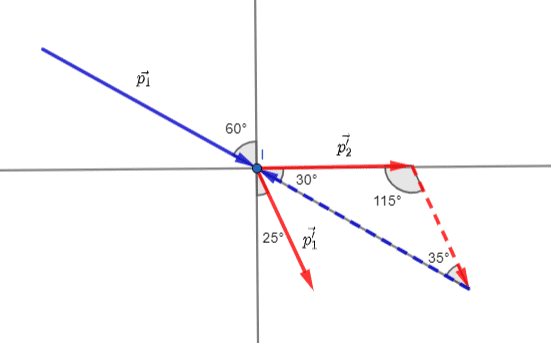# Momentum Collision Homework Problem -- help please

• lola1227

#### lola1227

Homework Statement
There is a situation in which M1=0.5kg, M2=0.5kg, V1 = 3m/s and V2=0. Find V1’ and V2’. I know we do component vectors and the we use the formula M1V1+M2v2=M1V1’+M2V2’ but I don’t know if we do perpendicular/parallel or vertical/horizontal and what we do with three angles?
Relevant Equations
M1V1+M2v2=M1V1’+M2V2’
Parallel:
M1V1+M2v2=M1V1’+M2V2’
(0.5)(3)+0=(0.5)(cos60)(3)+V2’Cos(x)(0.5)
V2’cos(x)=

Perpendicular:
M1V1+M2v2=M1V1’+M2V2’
0=(0.5)(0.3)(sin60)+V2’sin(x)(0.5)
V2’sin(x)=

And the divide 2 by 1

Which is tan(x)=2/1

And then plug then back into solve, but I don’t think we do it like this because we are only solving v2’ but we need v1’ as well?Can you post this "situation" exactly as it was given to you? It's probably a 2D collision but we need to know whether energy is conserved or not. Yes, conservation of momentum is applicable here. Usually, the direction of the motion is chosen the x-axis, but your drawing seems to indicate otherwise. We need to see the question as was posed to you and not your own interpretation of it because sometimes the two differ.

we need to know whether energy is conserved or not
I count two unknowns and there are two momentum equations available, so I think we can determine whether KE is conserved.

•Delta2
M1V1+M2v2=M1V1’+M2V2’
(0.5)(3)+0=(0.5)(cos60)(3)+V2’Cos(x)(0.5)
What happened to v1'? How come there is a velocity of 3 on the right, and how are you calculating the angle 60°?
0=(0.5)(0.3)(sin60)+V2’sin(x)(0.5)
Again, you seem to be assuming v1' is still 3m/s. And what is the value of x?

I count two unknowns and there are two momentum equations available, so I think we can determine whether KE is conserved.
I would say KE not conserved since if it were the post collision trajectories of the two equal mass objects would be at right angles.

I would begin by rotating the whole system anti-clockwise 40 degrees so that the angle for post collision momentum of ##m_2## is zero.

And then I would draw a vector diagram showing ##\vec{p_1'}+\vec{p_2'}-\vec{p_1}=0##

Last edited:
Can you post this "situation" exactly as it was given to you? It's probably a 2D collision but we need to know whether energy is conserved or not. Yes, conservation of momentum is applicable here. Usually, the direction of the motion is chosen the x-axis, but your drawing seems to indicate otherwise. We need to see the question as was posed to you and not your own interpretation of it because sometimes the two differ.
This is all that was given to us - and the drawing was given to us like this with these angles and everything

•kuruman
What happened to v1'? How come there is a velocity of 3 on the right, and how are you calculating the angle 60°?

Again, you seem to be assuming v1' is still 3m/s. And what is the value of x?
I meant to write COs theta which is the x and yeah I réalisés now I can’t use 3 for V1’

This is all that was given to us - and the drawing was given to us like this with these angles and everything
Yes, I understand now. Thanks for the clarification.

I would say KE not conserved since if it were the post collision trajectories of the two equal mass objects would be at right angles.

I would begin by rotating the whole system anti-clockwise 40 degrees so that the angle for post collision momentum of ##m_2## is zero.

And then I would draw a vector diagram showing ##\vec{p_1'}+\vec{p_2'}-\vec{p_1}=0##
We’re supposed to do it using vector components (horizontal/vertical) or paralllel/perpendicular but I’m not sure what to do or how to start it.

Yes, I understand now. Thanks for the clarification.
So, would we use the parallel/perpendicular or the vertical/horizontal and would we have two unknowns and plug one unknown into the other to solve them?

We’re supposed to do it using vector components (horizontal/vertical) or paralllel/perpendicular but I’m not sure what to do or how to start it.Sum of components blue and black = red. Not the easiest way perhaps - but it should get you there.

•Delta2
So, would we use the parallel/perpendicular or the vertical/horizontal and would we have two unknowns and plug one unknown into the other to solve them?
That's the idea. Formally, you would draw all vectors as arrows with their tails at the origin and then figure out the components. What @neilparker62 shows in post #12 is correct but could be confusing to a beginner because, for example, p1x is placed on the negative axis, yet it is a positive quantity since its arrow points to the right.

Last edited:
It seems to me that it is necessary to accept that the KE is not conserved or to clarify the terms with the one who made the task.

It seems to me that it is necessary to accept that the KE is not conserved or to clarify the terms with the one who made the task.
A good rule is not to assume conservation of mechanical energy without evidence for it. In the present case, there is enough information without it, so no need to think about whether it is nor any need for clarification.

•olsmukaHere I have implemented post #6. Since ##|\vec{p_1}|## is known , ##|\vec{p_1'}|## and ##|\vec{p_2'}|## - hence ##|\vec{v_1}'|## and ##|\vec{v_2}'|## - can be calculated using the sine rule.

I introduce two new axis : the collision normal axis n and the contact tangential axis t . Note that the initial velocities of both particles in the t axes must be zero. I hope this helps.